JEE  >  Test: 35 Year JEE Previous Year Questions: Simple Harmonic Motion (Oscillations)

# Test: 35 Year JEE Previous Year Questions: Simple Harmonic Motion (Oscillations)

Test Description

## 39 Questions MCQ Test Physics For JEE | Test: 35 Year JEE Previous Year Questions: Simple Harmonic Motion (Oscillations)

Test: 35 Year JEE Previous Year Questions: Simple Harmonic Motion (Oscillations) for JEE 2023 is part of Physics For JEE preparation. The Test: 35 Year JEE Previous Year Questions: Simple Harmonic Motion (Oscillations) questions and answers have been prepared according to the JEE exam syllabus.The Test: 35 Year JEE Previous Year Questions: Simple Harmonic Motion (Oscillations) MCQs are made for JEE 2023 Exam. Find important definitions, questions, notes, meanings, examples, exercises, MCQs and online tests for Test: 35 Year JEE Previous Year Questions: Simple Harmonic Motion (Oscillations) below.
Solutions of Test: 35 Year JEE Previous Year Questions: Simple Harmonic Motion (Oscillations) questions in English are available as part of our Physics For JEE for JEE & Test: 35 Year JEE Previous Year Questions: Simple Harmonic Motion (Oscillations) solutions in Hindi for Physics For JEE course. Download more important topics, notes, lectures and mock test series for JEE Exam by signing up for free. Attempt Test: 35 Year JEE Previous Year Questions: Simple Harmonic Motion (Oscillations) | 39 questions in 80 minutes | Mock test for JEE preparation | Free important questions MCQ to study Physics For JEE for JEE Exam | Download free PDF with solutions
 1 Crore+ students have signed up on EduRev. Have you?
Test: 35 Year JEE Previous Year Questions: Simple Harmonic Motion (Oscillations) - Question 1

### When a particle of mass m moves on the x-axis in a potential of the form V(x) = kx2 it performs simple harmonic motion. The corresponding time period is proportional to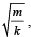as can be seen easily using dimensional analysis. However, the motion of a particle can be periodic even when its potential energy increases on both sides of x = 0 in a way different from kx2 and its total energy is such that the particle does not escape to infinity. Consider a particle of mass m moving on the x-axis. Its potential energy is V(x) = αx4 (α > 0) for |x| near the origin and becomes a constant equal to V0 for |x| > X0 (see figure).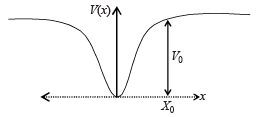Q. If the total energy of the particle is E, it will perform periodic motion only if

Detailed Solution for Test: 35 Year JEE Previous Year Questions: Simple Harmonic Motion (Oscillations) - Question 1

If the energy is zero, the particle will not perform oscillations. Therefore E should be greater than zero.
Further if E = V0, the potential energy will become constant as depicted in the graph given. In this case also the particle will not oscillate.
Therefore E should be greater than zero but less than V0.

Test: 35 Year JEE Previous Year Questions: Simple Harmonic Motion (Oscillations) - Question 2

### When a particle of mass m moves on the x-axis in a potential of the form V(x) = kx2 it performs simple harmonic motion. The corresponding time period is proportional toas can be seen easily using dimensional analysis. However, the motion of a particle can be periodic even when its potential energy increases on both sides of x = 0 in a way different from kx2 and its total energy is such that the particle does not escape to infinity. Consider a particle of mass m moving on the x-axis. Its potential energy is V(x) = αx4 (α > 0) for |x| near the origin and becomes a constant equal to V0 for |x| > X0 (see figure).Q. For periodic motion of small amplitude A, the time period T of this particle is proportional to

Detailed Solution for Test: 35 Year JEE Previous Year Questions: Simple Harmonic Motion (Oscillations) - Question 2

We can get the answer of this question with the help of dimensional analysis.

Given potential energy = αx4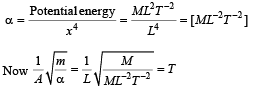Therefore option (b) is correct.

Test: 35 Year JEE Previous Year Questions: Simple Harmonic Motion (Oscillations) - Question 3

### When a particle of mass m moves on the x-axis in a potential of the form V(x) = kx2 it performs simple harmonic motion. The corresponding time period is proportional toas can be seen easily using dimensional analysis. However, the motion of a particle can be periodic even when its potential energy increases on both sides of x = 0 in a way different from kx2 and its total energy is such that the particle does not escape to infinity. Consider a particle of mass m moving on the x-axis. Its potential energy is V(x) = αx4 (α > 0) for |x| near the origin and becomes a constant equal to V0 for |x| > X0 (see figure).Q. The acceleration of this particle for |x| > X0 is

Detailed Solution for Test: 35 Year JEE Previous Year Questions: Simple Harmonic Motion (Oscillations) - Question 3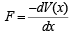As V (x) = constant for x > X0
∴ F = 0 for x > X0
Since F = 0, a = 0

Test: 35 Year JEE Previous Year Questions: Simple Harmonic Motion (Oscillations) - Question 4

Phase space diagrams are useful tools in analyzing all kinds of dynamical problems. They are especially useful in studying the changes in motion as initial position and momenum are changed.
Here we consider some simple dynamical systems in one dimension. For such systems, phase space is a plane in which position is plotted along horizontal axis and momentum is plotted along vertical axis. The phase space diagram is x(t) vs. p(t) curve in this plane. The arrow on the curve indicates the time flow. For example, the phase space diagram for a particle moving with constant velocity is a straight line as shown in the figure. We use the sign convention in which positon or momentum upwards (or to right) is positive and downwards (or to left) is negative.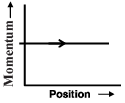Q. The phase space diagram for a ball thrown vertically up from ground is

Detailed Solution for Test: 35 Year JEE Previous Year Questions: Simple Harmonic Motion (Oscillations) - Question 4

When the ball is thrown upwards, at the point of throw (O) the linear momentum is in upwards direction (and has a maximum value ) and the position is zero. As the time passes, the ball moves upwards and its momentum goes on decreasing and the position becomes positive. The momentum becomes zero at the topmost point (A).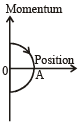As the time increases, the ball starts moving down with an increasing linear momentum in the downward direction (negative) and reaches back to its original position.
These characteristics are represented by graph (d).

Test: 35 Year JEE Previous Year Questions: Simple Harmonic Motion (Oscillations) - Question 5

Phase space diagrams are useful tools in analyzing all kinds of dynamical problems. They are especially useful in studying the changes in motion as initial position and momenum are changed.
Here we consider some simple dynamical systems in one dimension. For such systems, phase space is a plane in which position is plotted along horizontal axis and momentum is plotted along vertical axis. The phase space diagram is x(t) vs. p(t) curve in this plane. The arrow on the curve indicates the time flow. For example, the phase space diagram for a particle moving with constant velocity is a straight line as shown in the figure. We use the sign convention in which positon or momentum upwards (or to right) is positive and downwards (or to left) is negative.Q. The phase space diagram for simple harmonic motion is a circle centered at the origin. In the figure, the two circles represent the same oscillator but for different initial conditions, and E1 and E2 are the total mechanical energies respectively. Then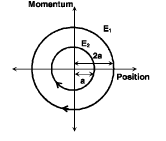Detailed Solution for Test: 35 Year JEE Previous Year Questions: Simple Harmonic Motion (Oscillations) - Question 5

We know that the total mechanical energy ∝ (Amplitude)2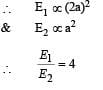Test: 35 Year JEE Previous Year Questions: Simple Harmonic Motion (Oscillations) - Question 6

Phase space diagrams are useful tools in analyzing all kinds of dynamical problems. They are especially useful in studying the changes in motion as initial position and momenum are changed.
Here we consider some simple dynamical systems in one dimension. For such systems, phase space is a plane in which position is plotted along horizontal axis and momentum is plotted along vertical axis. The phase space diagram is x(t) vs. p(t) curve in this plane. The arrow on the curve indicates the time flow. For example, the phase space diagram for a particle moving with constant velocity is a straight line as shown in the figure. We use the sign convention in which positon or momentum upwards (or to right) is positive and downwards (or to left) is negative.Q. Consider the spring-mass system, with the mass submerged in water, as shown in the figure. The phase space diagram for one cycle of this system is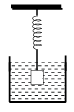Detailed Solution for Test: 35 Year JEE Previous Year Questions: Simple Harmonic Motion (Oscillations) - Question 6

As the mass is osicillating in water its amplitude will go on decreasing and the amplitude will decrease with time. Options (c) and (d) cannot be true.
When the position of the mass is at one extreme end in the positive side (the topmost point), the momentum is zero. As the mass moves towards the mean position the momentum increases in the negative direction.
These characteristics are depicted in option (b).

Test: 35 Year JEE Previous Year Questions: Simple Harmonic Motion (Oscillations) - Question 7

In a simple harmonic oscillator, at the mean position

Detailed Solution for Test: 35 Year JEE Previous Year Questions: Simple Harmonic Motion (Oscillations) - Question 7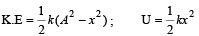At the mean position x = 0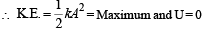Test: 35 Year JEE Previous Year Questions: Simple Harmonic Motion (Oscillations) - Question 8

If a spring has time period T, and is cut into n equal parts, then the time period of each part will be

Detailed Solution for Test: 35 Year JEE Previous Year Questions: Simple Harmonic Motion (Oscillations) - Question 8

Let the spring constant of the original spring be k.
Then its time period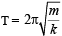where m is the mass of oscillating body.
When the spring is cut into n equal parts, the spring constant of one part becomes nk. Therefore the new time period,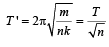Test: 35 Year JEE Previous Year Questions: Simple Harmonic Motion (Oscillations) - Question 9

A child swinging on a swing in sitting position, stands up, then the time period of the swing will

Detailed Solution for Test: 35 Year JEE Previous Year Questions: Simple Harmonic Motion (Oscillations) - Question 9

Let the spring constant of the original spring be k. Then its time period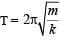where m is the mass of
oscillating body.
When the spring is cut into n equal parts, the spring constant of one part becomes nk. Therefore the new time period,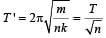Test: 35 Year JEE Previous Year Questions: Simple Harmonic Motion (Oscillations) - Question 10

A mass M is suspended from a spring of negligible mass. The spring is pulled a little and then released so that the mass executes SHM of  time period T. If the mass is increased by m, the time period becomes 5T/3.  Then the ratio of  m/M is

Detailed Solution for Test: 35 Year JEE Previous Year Questions: Simple Harmonic Motion (Oscillations) - Question 10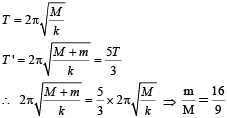Test: 35 Year JEE Previous Year Questions: Simple Harmonic Motion (Oscillations) - Question 11

Two particles A and B of equal masses are suspended from two massless springs of spring of spring constant k1 and k2, respectively. If the maximum velocities, during oscillation, are equal, the ratio of amplitude of  A and B is

Detailed Solution for Test: 35 Year JEE Previous Year Questions: Simple Harmonic Motion (Oscillations) - Question 11

Maximum velocity during SHM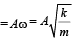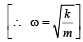Here the maximum velocity is same and m is also same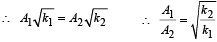Test: 35 Year JEE Previous Year Questions: Simple Harmonic Motion (Oscillations) - Question 12

The length of a simple pendulum executing simple harmonic motion is increased by 21%. The percentage increase in the time period of the pendulum of  increased length is

Detailed Solution for Test: 35 Year JEE Previous Year Questions: Simple Harmonic Motion (Oscillations) - Question 12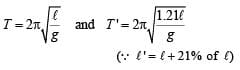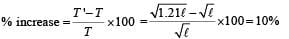Test: 35 Year JEE Previous Year Questions: Simple Harmonic Motion (Oscillations) - Question 13

The displacement of a particle varies according to the relation x = 4(cos πt + sinπt). The amplitude of the particle is

Detailed Solution for Test: 35 Year JEE Previous Year Questions: Simple Harmonic Motion (Oscillations) - Question 13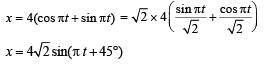Test: 35 Year JEE Previous Year Questions: Simple Harmonic Motion (Oscillations) - Question 14

A body executes simple harmonic motion. The potential energy (P.E), the kinetic energy (K.E) and total energy (T.E) are measured as a function of displacement x. Which of the following statements is true ?

Detailed Solution for Test: 35 Year JEE Previous Year Questions: Simple Harmonic Motion (Oscillations) - Question 14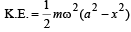When x = 0,  K.E is maximum and is equal to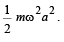Test: 35 Year JEE Previous Year Questions: Simple Harmonic Motion (Oscillations) - Question 15

The bob of a simple pendulum executes simple harmonic motion in water with a period t, while the period of oscillation of the bob is t0 in air. Neglecting frictional force of water and given that the density of the bob is (4 / 3) x 1000 kg/m3 .
What relationship between t and t0 is true

Detailed Solution for Test: 35 Year JEE Previous Year Questions: Simple Harmonic Motion (Oscillations) - Question 15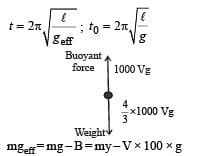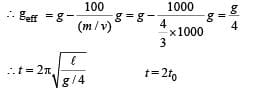Test: 35 Year JEE Previous Year Questions: Simple Harmonic Motion (Oscillations) - Question 16

A particle at the end of a spring executes S.H.M with a period t1. while the corresponding period for another spring is t2. If the period of oscillation with the two springs in series is T then

Detailed Solution for Test: 35 Year JEE Previous Year Questions: Simple Harmonic Motion (Oscillations) - Question 16

For first spring,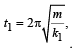For second spring,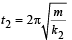when springs are in series then,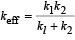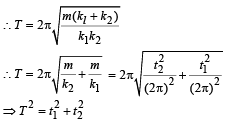where x is the displacement from the mean position

Test: 35 Year JEE Previous Year Questions: Simple Harmonic Motion (Oscillations) - Question 17

The total energy of a particle, executing simple harmonic motion is where x is the displacement from the mean position, hence total energy is independent of x.

Detailed Solution for Test: 35 Year JEE Previous Year Questions: Simple Harmonic Motion (Oscillations) - Question 17

At any instant the total  energy is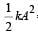= constant,   where  A = amplitude
hence total energy is independent of x.

Test: 35 Year JEE Previous Year Questions: Simple Harmonic Motion (Oscillations) - Question 18

A particle of mass m is attached to a spring (of spring constant k) and has a natural angular frequency ω0. An external force F(t) pr opor tion al to cos ωt (ω ≠ ω0) is applied to th e oscillator. The time displacement of the oscillator will be proportional to

Detailed Solution for Test: 35 Year JEE Previous Year Questions: Simple Harmonic Motion (Oscillations) - Question 18

Equation of displacement is given by

x = A sin(ωt +φ)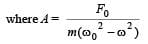Test: 35 Year JEE Previous Year Questions: Simple Harmonic Motion (Oscillations) - Question 19

In forced oscillation of a particle the amplitude is maximum for a frequency ω1 of the force while the energy is maximum for a frequency ω2 of the force; then

Detailed Solution for Test: 35 Year JEE Previous Year Questions: Simple Harmonic Motion (Oscillations) - Question 19

The maximum of amplitude and energy is obtained when the frequency is equal to the natural frequency (resonance condition)
∴ w1 = w2

Test: 35 Year JEE Previous Year Questions: Simple Harmonic Motion (Oscillations) - Question 20

Two simple harmonic motions are represented by the equations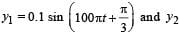= 0.1 cos πt . The phase difference of the velocity of particle 1 with respect to the velocity of particle 2 is

Detailed Solution for Test: 35 Year JEE Previous Year Questions: Simple Harmonic Motion (Oscillations) - Question 20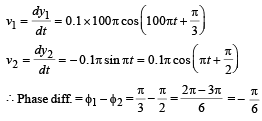Test: 35 Year JEE Previous Year Questions: Simple Harmonic Motion (Oscillations) - Question 21

The function sin2 (ωt) represents

Detailed Solution for Test: 35 Year JEE Previous Year Questions: Simple Harmonic Motion (Oscillations) - Question 21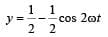Test: 35 Year JEE Previous Year Questions: Simple Harmonic Motion (Oscillations) - Question 22

The bob of a simple pendulum is a spherical hollow ball filled with water. A plugged hole near the bottom of the oscillating bob gets suddenly unplugged. During observation, till water is coming out, the time period of oscillation would

Detailed Solution for Test: 35 Year JEE Previous Year Questions: Simple Harmonic Motion (Oscillations) - Question 22

Centre of mass of combination of liquid and hollow portion (at position ℓ), first goes down (to ℓ + Δℓ) and when total water is drained out, centre of mass regain its original position (to ℓ),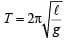∴ ‘T’ first increases and then decreases to original value.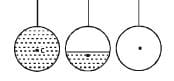Test: 35 Year JEE Previous Year Questions: Simple Harmonic Motion (Oscillations) - Question 23

If a simple harmonic motion is represented by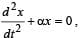its time period is

Detailed Solution for Test: 35 Year JEE Previous Year Questions: Simple Harmonic Motion (Oscillations) - Question 23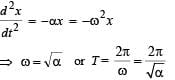Test: 35 Year JEE Previous Year Questions: Simple Harmonic Motion (Oscillations) - Question 24

Th e maximum velocity of a particle, executin g simple harmonic motion with an amplitude 7 mm, is 4.4 m/s. The period of oscillation is

Detailed Solution for Test: 35 Year JEE Previous Year Questions: Simple Harmonic Motion (Oscillations) - Question 24

Maximum velocity,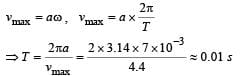Test: 35 Year JEE Previous Year Questions: Simple Harmonic Motion (Oscillations) - Question 25

Starting from the origin a body oscillates simple harmonically with a period of 2 s. After what time will its kinetic energy be 75% of the total energy?

Detailed Solution for Test: 35 Year JEE Previous Year Questions: Simple Harmonic Motion (Oscillations) - Question 25

K.E. of a body undergoing SHM is given by,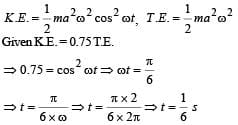Test: 35 Year JEE Previous Year Questions: Simple Harmonic Motion (Oscillations) - Question 26

Two springs, of force constants k1 and k2 are connected to a mass m as shown. The frequency of oscillation of the mass is f. If both k1 and k2 are made four times their original values, the frequency of oscillation becomes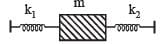Detailed Solution for Test: 35 Year JEE Previous Year Questions: Simple Harmonic Motion (Oscillations) - Question 26

The two springs are in parallel.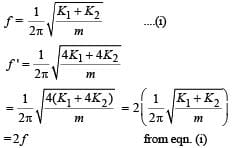Test: 35 Year JEE Previous Year Questions: Simple Harmonic Motion (Oscillations) - Question 27

A particle of mass m executes simple harmonic motion with amplitude a and frequency v. The average kinetic energy during its motion from the position of equilibrium to the end is

Detailed Solution for Test: 35 Year JEE Previous Year Questions: Simple Harmonic Motion (Oscillations) - Question 27

(b) KEY CONCEPT : The instantaneous kinetic energy of a particle executing S.H.M. is given by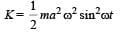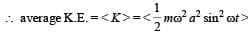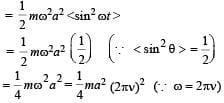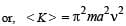Test: 35 Year JEE Previous Year Questions: Simple Harmonic Motion (Oscillations) - Question 28

The displacement of an object attached to a spring and executing simple harmonic motion is given by x = 2 × 10–2 cos πt metre.The time at which the maximum speed first occurs is

Detailed Solution for Test: 35 Year JEE Previous Year Questions: Simple Harmonic Motion (Oscillations) - Question 28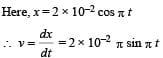For the first time, the speed to be maximum,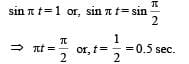Test: 35 Year JEE Previous Year Questions: Simple Harmonic Motion (Oscillations) - Question 29

A point mass oscillates along the x-axis according to the law x = x0 cos(ωt -π / 4) . If the acceleration of the particle is written as a = A cos(wt +δ) ,then

Detailed Solution for Test: 35 Year JEE Previous Year Questions: Simple Harmonic Motion (Oscillations) - Question 29

Here,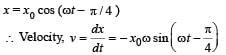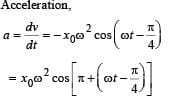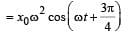...(1)
Acceleration, a = A cos (ωt + δ) ...(2)
Comparing the two equations, we get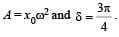Test: 35 Year JEE Previous Year Questions: Simple Harmonic Motion (Oscillations) - Question 30

If x, v and a denote the displacement, the velocity and the acceleration of a particle executing simple harmonic motion of time period T, then, which of the following does not change with time?

Detailed Solution for Test: 35 Year JEE Previous Year Questions: Simple Harmonic Motion (Oscillations) - Question 30

For an SHM, the acceleration a = -ω2x where ω2 is a constant. Therefore a/x  is a constant. The time period
T is also constant. Therefor aT/x is a constant.

Test: 35 Year JEE Previous Year Questions: Simple Harmonic Motion (Oscillations) - Question 31

Two particles are executing simple harmonic motion of the same amplitude A and frequency ω along the x-axis. Their mean position is separated by distance X0(X0 > A). If the maximum separation between them is (X0 + A), the phase difference between their motion is:

Detailed Solution for Test: 35 Year JEE Previous Year Questions: Simple Harmonic Motion (Oscillations) - Question 31

For X0 + A to be the maximum seperation y one body is at the mean position, the other should be at the extreme.

Test: 35 Year JEE Previous Year Questions: Simple Harmonic Motion (Oscillations) - Question 32

A mass M, attached to a horizontal spring, executes S.H.M. with amplitude A1. When the mass M passes through its mean position then a smaller mass m is placed over it and both of them move together with amplitude A2. The ratio of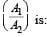Detailed Solution for Test: 35 Year JEE Previous Year Questions: Simple Harmonic Motion (Oscillations) - Question 32

The net force becomes zero at the mean point.
Therefore, linear momentum must be conserved.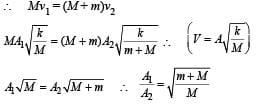Test: 35 Year JEE Previous Year Questions: Simple Harmonic Motion (Oscillations) - Question 33

If a simple pendulum has significant amplitude (up to a factor of 1/e of original) only in the period between t = 0s to t = τ s, then τ may be called the average life of the pendulum.
When the spherical bob of the pendulum suffers a retardation (due to viscous drag) proportional to its velocity with b as the constant of proportionality, the average life time of the pendulum is (assuming damping is small) in seconds :

Detailed Solution for Test: 35 Year JEE Previous Year Questions: Simple Harmonic Motion (Oscillations) - Question 33

The equation of motion for the pendulum, suffering retardation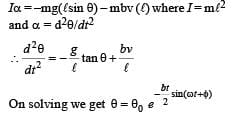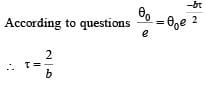Test: 35 Year JEE Previous Year Questions: Simple Harmonic Motion (Oscillations) - Question 34

The amplitude of a damped oscillator decreases to 0.9 times its original magnitude in 5s. In another 10s it will decrease to a times its original magnitude, where α equals

Detailed Solution for Test: 35 Year JEE Previous Year Questions: Simple Harmonic Motion (Oscillations) - Question 34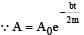(where, A0  = maximum amplitude)
According to the questions, after 5 second,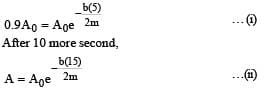From eqns (i) and (ii)
A = 0.729 A0 ∴ α = 0.729

Test: 35 Year JEE Previous Year Questions: Simple Harmonic Motion (Oscillations) - Question 35

An ideal gas enclosed in a vertical cylindrical container supports a freely moving piston of mass M. The piston and the cylinder have equal cross sectional area A. When the piston is in equilibrium, the volume of the gas is V0 and its pressure is P0. The piston is slightly displaced from the equilibrium position and released. Assuming that the system is completely isolated from its surrounding, the piston executes a simple harmonic motion with frequency

Detailed Solution for Test: 35 Year JEE Previous Year Questions: Simple Harmonic Motion (Oscillations) - Question 35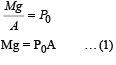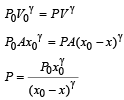Let piston is displaced by distance x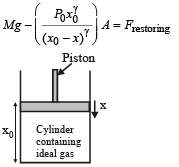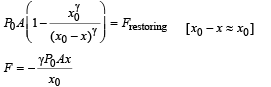∴  Frequency with which piston executes SHM.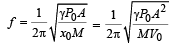Test: 35 Year JEE Previous Year Questions: Simple Harmonic Motion (Oscillations) - Question 36

A particle moves with simple harmonic motion in a straight line. In first τ s, after starting from rest it travels a distance a, and in next τ s it travels 2a, in same direction, then:

Detailed Solution for Test: 35 Year JEE Previous Year Questions: Simple Harmonic Motion (Oscillations) - Question 36

In simple harmonic motion, starting from rest,

At t = 0 , x = A
x = Acosωt ..... (i)
When t = τ , x = A – a
When t = 2 τ , x = A –3a
From equation (i)
A – a = Acosω τ ......(ii)
A – 3a = A cos2ω τ ......(iii)
As cos2ωτ = 2 cos2 ωτ – 1 …(iv)

From equation (ii), (iii)  and (iv)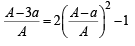|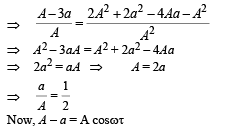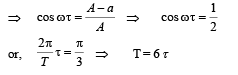Test: 35 Year JEE Previous Year Questions: Simple Harmonic Motion (Oscillations) - Question 37

A pendulum made of a uniform wire of cross sectional area A has time period T. When an additional mass M is added to its bob, the time period changes to TM. If the Young's modulus of the material of the wire is Y then 1/Y is equal to :

(g = gravitational acceleration)

Detailed Solution for Test: 35 Year JEE Previous Year Questions: Simple Harmonic Motion (Oscillations) - Question 37

As we know, time period,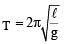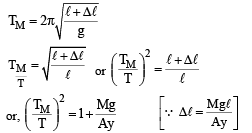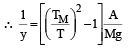Test: 35 Year JEE Previous Year Questions: Simple Harmonic Motion (Oscillations) - Question 38

For a simple pendulum, a graph is plotted between its kinetic energy (KE) and potential energy (PE) against its displacement d.
Which one of the following represents these correctly? (graphs are schematic and not drawn to scale)

Detailed Solution for Test: 35 Year JEE Previous Year Questions: Simple Harmonic Motion (Oscillations) - Question 38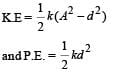At mean position  d = 0. At extremes positions d = A

Test: 35 Year JEE Previous Year Questions: Simple Harmonic Motion (Oscillations) - Question 39

A particle performs simple harmonic mition with amplitude A. Its speed is trebled at the instant that it is at a distance 2A/3 from equilibrium position. The new amplitude of themotion is :

Detailed Solution for Test: 35 Year JEE Previous Year Questions: Simple Harmonic Motion (Oscillations) - Question 39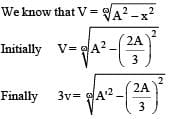Where A'= final amplitude (Given at x = 2A/3, velocity
to trebled)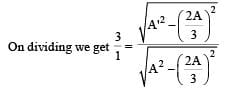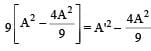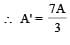## Physics For JEE

258 videos|633 docs|256 tests
 Use Code STAYHOME200 and get INR 200 additional OFF Use Coupon Code
Information about Test: 35 Year JEE Previous Year Questions: Simple Harmonic Motion (Oscillations) Page
In this test you can find the Exam questions for Test: 35 Year JEE Previous Year Questions: Simple Harmonic Motion (Oscillations) solved & explained in the simplest way possible. Besides giving Questions and answers for Test: 35 Year JEE Previous Year Questions: Simple Harmonic Motion (Oscillations), EduRev gives you an ample number of Online tests for practice

## Physics For JEE

258 videos|633 docs|256 tests